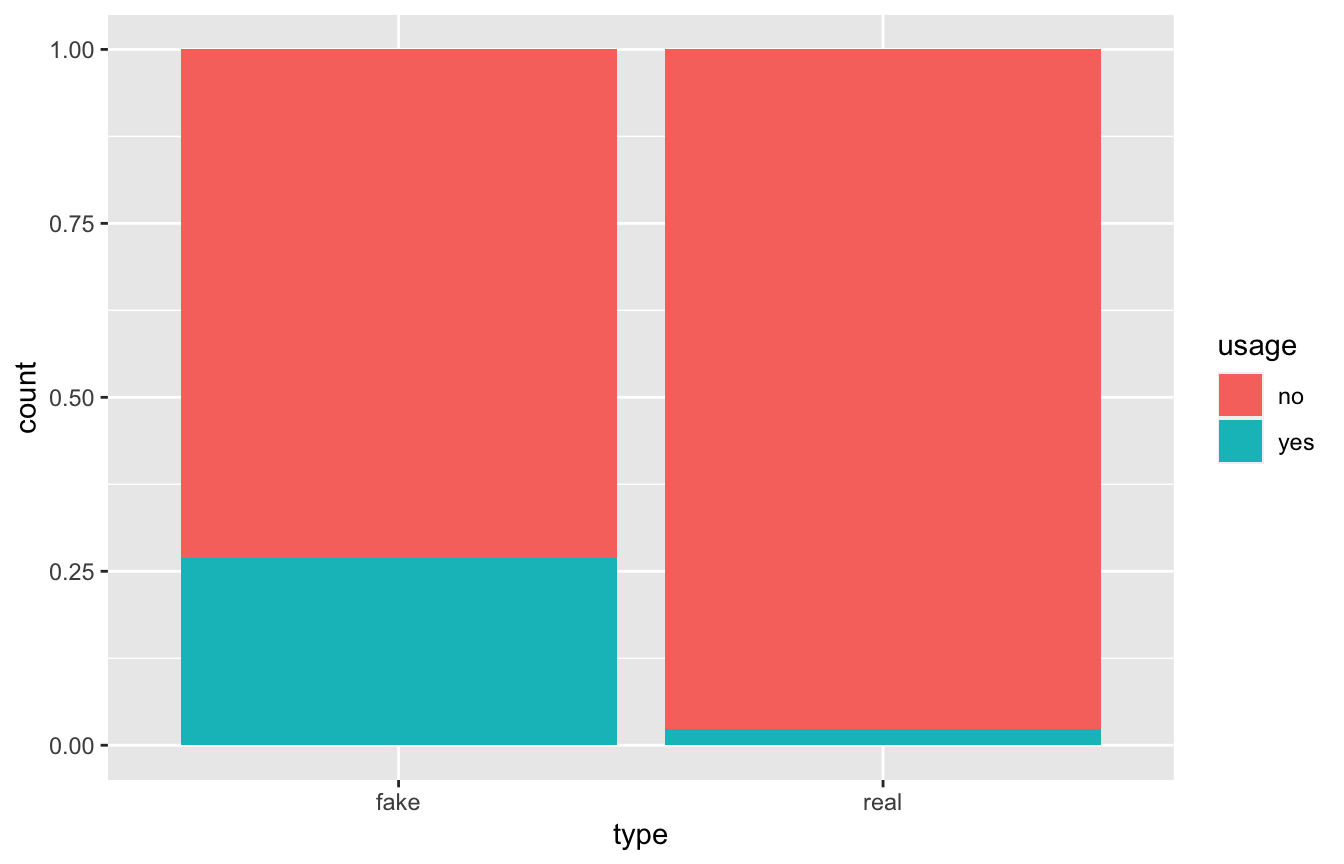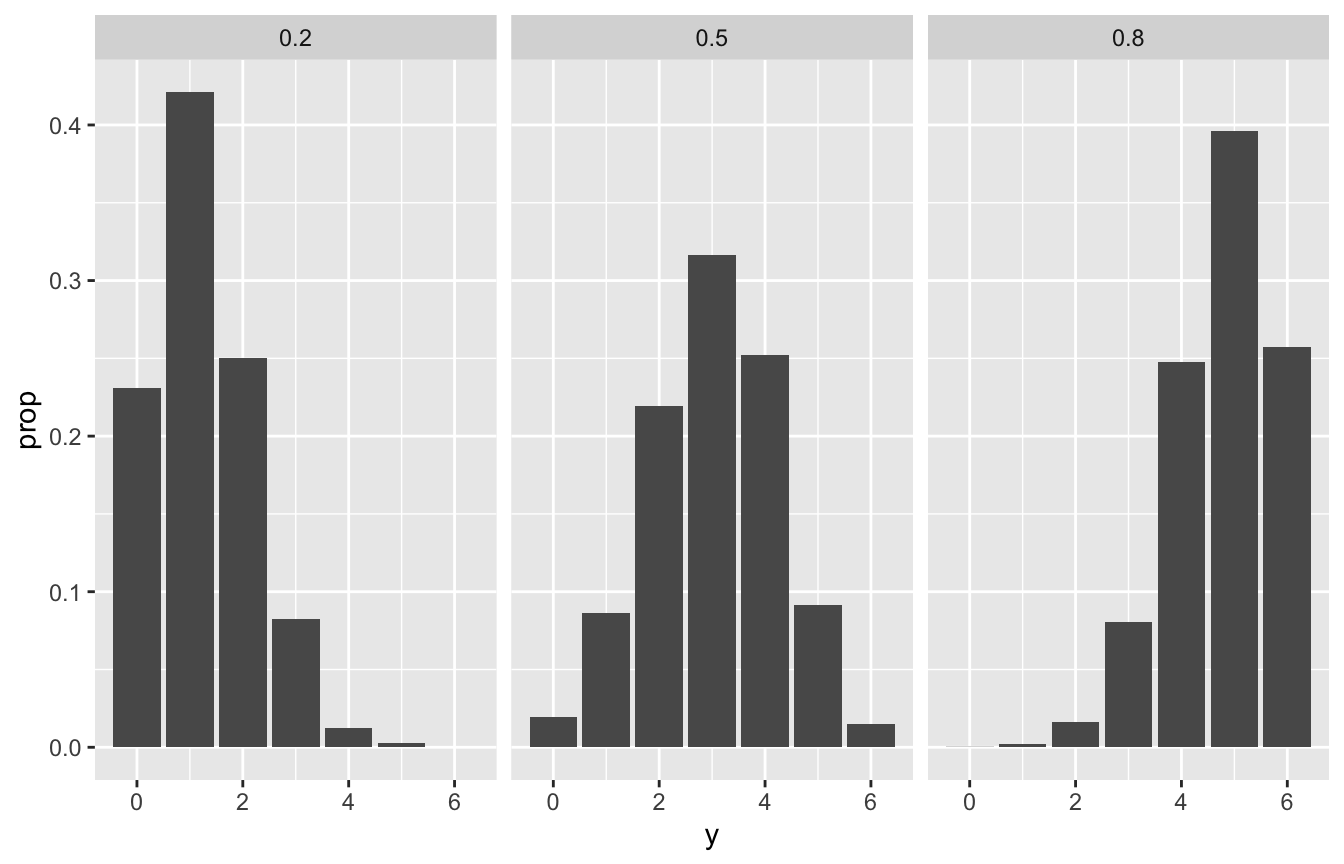Published

September 3, 2022

# Load packages
library(bayesrules)
library(tidyverse)
library(janitor)

# Import article data
data(fake_news)

perc <- scales::label_percent(accuracy = 1)
perc2 <- scales::label_percent(accuracy = 0.01)

How many are fake vs. real?

fake_news %>%
count(type) %>%
##   type   n
##   fake  60
##   real  90
##  Total 150

60/150 or 40% of news articles are fake .

How is the use of exclamation marks distributed across fake and real news articles?

fake_news %>%
tabyl(title_has_excl, type) %>%
##  title_has_excl fake real
##           FALSE   44   88
##            TRUE   16    2
##           Total   60   90

16/60 or 26.67% of news articles with !s are fake; only 2/90 or 2.22% of real articles with have !s.

Our prior is thus that 40% of news articles are fake. We have new data, that !s are more common in fake news articles. So what’s the posterior if we find an article with a !?

The chance that this article is fake jumps from 40% to roughly 90%. Though exclamation points are more common among fake articles, let’s not forget that only 40% of articles are fake.

## Conditional probabilities

We have two variables:

• Fake vs. real status
• Use of exclamation points

These features can vary at random across different articles, so we have to represent that randomness with probabilities models

### Prior probability model

We know from previous data that 40% are fake and 60% are real. If $$B$$ means the article is fake, we can write that as

$P(B) = 0.40 \text{ and } P(B^c) = 0.60$

### Conditional probability

The occurrence of !s depends on fakeness. Conditional probabilities of !s and fakeness, where $$A$$ is the use of an exclamation mark:

$P(A \mid B) = 0.2667 \text{ and } P(A \mid B^c) = 0.0222$

By comparing conditional vs. unconditional probabilities, we learn how much $$B$$ can inform our understanding of $$A$$.

An event $$A$$ might increase in probability given $$B$$, like how the probability of joining an orchestra is greater if we know someonw practices daily:

$P(\text{join orchestra} \mid \text{practice daily}) > P(\text{join orchestra})$

Or the probability of getting the flu is lower if you know someone washes their hands a lot:

$P(\text{get flu} \mid \text{wash hands regularly}) < P(\text{get flu})$

### Likelihood

Likelihood is kind of like the inverse of probability (not really! just that the order of A and B matters)

• If we know $$B$$, the conditional probability $$P(\cdot \mid B)$$ lets us compare the probabilities of an unknown event $$A$$ (or $$A^c$$) occurring with $$B$$, or

$P(A \mid B) \text{ vs. } P(A^c \mid B)$

• If we know $$A$$, the likelihood function $$L(\cdot \mid A) = P(A \mid \cdot)$$ lets us compare the relative compatibility of data $$A$$ with events $$B$$ or $$B^c$$

$L(B \mid A) \text{ vs. } L(B^c \mid A)$

What this looks like in practice, where $$A$$ means having an exclamation mark and $$B$$ means being fake:

# Prior probability
row_prior <- fake_news %>%
count(type) %>%
mutate(prop = n / sum(n)) %>%
select(-n) %>%
pivot_wider(names_from = type, values_from = prop)

# Likelihood
row_likelihood <- fake_news %>%
count(type, title_has_excl) %>%
pivot_wider(names_from = title_has_excl, values_from = n) %>%
mutate(likelihood = TRUE / (TRUE + FALSE)) %>%
select(-c(FALSE, TRUE)) %>%
pivot_wider(names_from = type, values_from = likelihood)

bind_cols(Statistic = c("Prior probability", "Likelihood"),
bind_rows(row_prior, row_likelihood)) %>%
mutate(Total = fake + real) %>%
rename(Fake ($B$) = fake,
Real ($B^c$) = real) %>%
knitr::kable(digits = 3)
Statistic Fake ($$B$$) Real ($$B^c$$) Total
Prior probability 0.400 0.600 1.000
Likelihood 0.267 0.022 0.289

### Normalizing constants

The last piece we need is the marginal probability of observing exclamation points across all articles, or $$P(A)$$, which is the normalizing constant, or $$P(B)L(B \mid A) + P(B^c)L(B^c \mid A)$$

fake_news %>%
count(type, title_has_excl) %>%
mutate(prop = n / sum(n)) %>%
filter(title_has_excl == TRUE) %>%
summarize(normalizing_constant = sum(prop))
##   normalizing_constant
## 1                 0.12

### Final analytical posterior

Thus, given this formula:

$\text{posterior} = \frac{\text{prior} \times \text{likelihood}}{\text{normalizing constant}}$

…we have

$\text{posterior} = \frac{0.4 \times 0.2667}{0.12} = 0.889$

## Simulation

We can simulate this too

sim_params <- tibble(type = c("real", "fake"),
prior = c(0.6, 0.4))

set.seed(1234)

sims <- sample(sim_params$type, size = 10000, prob = sim_params$prior, replace = TRUE) %>%
enframe(value = "type") %>%
mutate(data_model = case_when(type == "fake" ~ 0.2667,
type == "real" ~ 0.0222)) %>%
rowwise() %>%
mutate(usage = sample(c("no", "yes"), size = 1,
prob = c(1 - data_model, data_model))) %>%
ungroup()

sims %>%
tabyl(usage, type) %>%
##  usage fake real Total
##     no 2914 5872  8786
##    yes 1075  139  1214
##  Total 3989 6011 10000

ggplot(sims, aes(x = type, fill = usage)) +
geom_bar(position = position_fill())# Posterior
sims %>%
filter(usage == "yes") %>%
count(type) %>%
mutate(prop = n / sum(n))
## # A tibble: 2 × 3
##   type      n  prop
##   <chr> <int> <dbl>
## 1 fake   1075 0.886
## 2 real    139 0.114

## Chess simulation

chess <- c(0.2, 0.5, 0.8)
prior <- c(0.1, 0.25, 0.65)

set.seed(1234)
chess_sim <- tibble(pi = sample(chess, size = 10000, prob = prior, replace = TRUE)) %>%
mutate(y = rbinom(n(), size = 6, prob = pi))

chess_sim %>%
count(pi) %>%
mutate(prop = n / sum(n))
## # A tibble: 3 × 3
##      pi     n   prop
##   <dbl> <int>  <dbl>
## 1   0.2   986 0.0986
## 2   0.5  2523 0.252
## 3   0.8  6491 0.649

chess_sim %>%
ggplot(aes(x = y)) +
stat_count(aes(y = ..prop..)) +
facet_wrap(vars(pi))
## Warning: The dot-dot notation (..prop..) was deprecated in ggplot2 3.4.0.
## ℹ Please use after_stat(prop) instead.